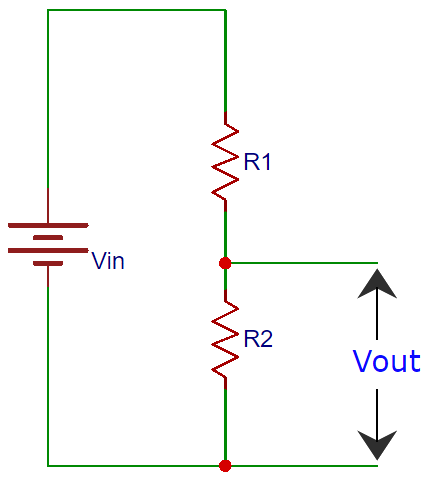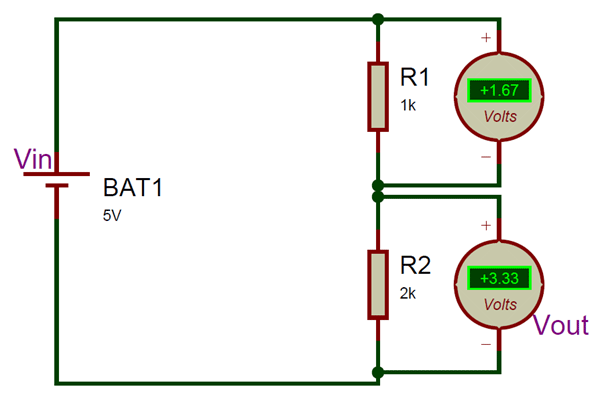# Voltage Divider CalculatorPlease see the voltage divider circuit provided here and calculate the output voltage using voltage divider calculator according to the following voltage divider formula:

Vout= (Vin x R2) / (R1 + R2)

Here:

• Vin is the input voltage
• R1 is the resistance of the 1st resistor,
• R2 is the resistance of the 2nd resistor,
• Vout is the output voltage.

Alternatively, you can also use this voltage divider calculator to provide any 3 known values in the circuit and calculate the 4th one.

 Input Voltage (Vin): Volt Resistance 1 (R1) Ohm Kilo Ohm Mega Ohm Resistance 2 (R2) Ohm Kilo Ohm Mega Ohm Output Voltage (Vout): Volt

A potential divider circuit is a very common circuit used in electronics where an input voltage has to be converted to another voltage less than it. This circuit is very useful for all analog circuits where variable voltages are required, hence it is important to understand how this circuit works and how to calculate the values of Resistors.

A voltage divider circuit is very simple circuit consisting of only two resistors (R1 and R2) as shown above. The required output voltage (Vout) can be obtained across the resistor R2.  Using these two resistors we can convert an input voltage to any required output voltage, this output voltage is decided by the value of the resistance R1 and R2. The formulae to calculate Vout is shown below.

Vout= (Vin x R2) / (R1 + R2)

Where, Vout= Output Voltage Vin=Input Voltage and R1=Upper Resistor R2=Lower resistor

We can use the above voltage divider calculator to calculate any one of the value mentioned in the voltage divider formulae, but now let us learn how this formulae was derived. Consider the below circuit which can be used to convert 5V input to 3.3V output for analysisTo understand how a potential diver formulae is derived we need Ohms law calculator , according to Ohms law the voltage drop across any location is a product of the current flowing through the circuit and the Resistance across it.

Voltage = Current Flowing through × Resistance across the voltage

Let us use this to calculate the Input Voltage (Vin) for the above circuit. Here there are two resistors across input voltage Vin, hence

Input Voltage = Current × (Resistance 1 + Resistance 2)

Vin = I × (R1 + R2)     (1)

Similarly let us calculate for output voltage (Vout), here there is only one resistor (R2) across it hence

Output Voltage = Current × Resistance R2

Vout  = I × R2             (2)

If we look at the equation 1 and 2 we can notice that the value of current is same, hence lets re-write

Equation 1 as,   I = Vin / (R1 + R2)

Equation 2 as, I = Vout /R2

Since the current flowing through the circuit is constant, the current I will remain same for both the equations hence we can equate them as

Vin / (R1 + R2) = Vout /R2

Vout= (Vin x R2) / (R1 + R2)

Let us test this voltage divider formulae for the above circuit where Vin =5V, R1 = 1000ohms and R2 = 2000ohms.

Vout  = (5×2000) / (1000/2000)

Vout  = (10000) / (3000)

Vout  = 3.3333V

Another important factor to consider while selecting the resistor values is its power rating (P). Once you know the values of I (based in load), Vin, R1 and R2, add R1 and R2 together to get RTOTAL and use the Ohms law calculator to find out the power(Watts) rating required for the resistors. Or simply use the formulae P=VI to decide on the power rating for your resistor. If a proper Power rating is not selected the resistor will overheat and might also burn.